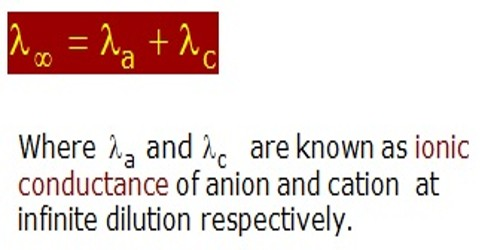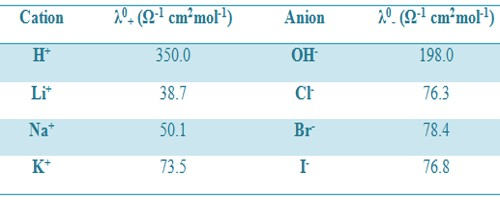Chemistry

# Independent Migration of Ions: Kohlrausch’s LawIndependent Migration of Ions: Kohlrausch’s Law

In 1874, Kohlrausch formulated the law of independent migration of ions based on the experimental data of conductivities of various electrolytes. This law can be stated as follows:

At infinite dilution, the dissociation of the electrolyte is complete and hence each ion makes definite contribution to the equivalent conductivity of the electrolyte irrespective of the nature of other ions associated with it.

Kohlrausch examined the values of Λ0m of a number of strong electrolytes with common anions or cations and found some regularity in the Λ0m values of various electrolytes. He observed that when the electrolytes were considered in pairs having one common ion the difference between the Λ0m values were constant (Table).

Table: Differences in values of Λ0m for several pairs of electrolytes with a common ion.These observations led Kohlrausch (1875) to state that the molar conductance at infinite dilution is different for different electrolytes and is equal to the sum of the conductance of the constituent ions of the electrolytes. This is known as the Kohlrausch’s law of independent ionic migration. The law may be expressed in the form

Λ0m = λ0+ + λ0

Where λ0+ and λ0 are called the ion conductance of the cation and anion respectively. The above equation means that each ion of an electrolyte contributes independently to the value of Λ0m at infinite dilution. For example, the molar conductance of KCl at infinite dilution is 149.86 Ω-1 cm2mol-1. The ion conductance’s of K+ and Cl are 73.50 and 76.30 Ω-1 cm2mol-1. According to Kohlrausch law independent ionic migration,

Λ0m(KCl) = λ0K+ + λ0Cl-

= 73.50 + 76.30 Ω-1 cm2mol-1

= 149.80 Ω-1 cm2mol-1

this is in agreement with the value measured directly. The Kohlrausch’s law of independent ionic migration is useful for calculation of Λ° for weak electrolytes for which Λ0m cannot be ascertained from the plot of Λm vs √C as shown in Table.

Table: Ion conductance at 25°CThe computation is done by addition and subtraction of Λ0 values for different strong electrolysis.